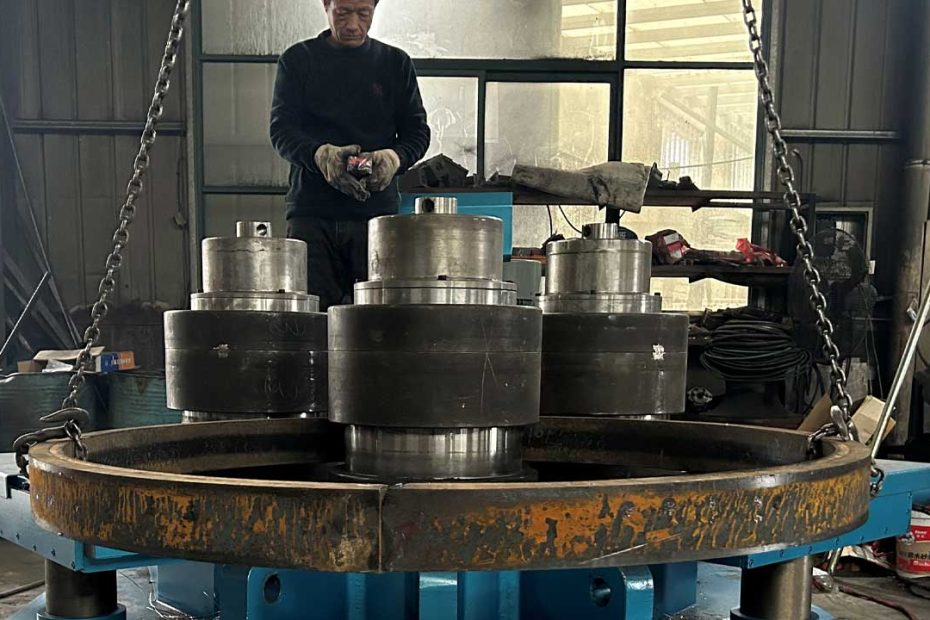# How is section modulus used in section bending processes?To determine if a steel section can be curved you need to first determine its section modulus and then see if you have the correct bending equipment.

Section modulus is the direct measure of the strength of the steel. Bending a steel section that has a larger section modulus than another will be stronger and harder to bend. Section modulus is a geometric property for a given cross-section used in the design of flexural members.

There are two types of section moduli, the elastic section modulus (S) and the plastic section modulus (Z).

Another property used in beam design is section modulus (Z).

The section modulus of the cross-sectional shape is of significant importance in designing beams. It is a direct measure of the strength of the beam. A beam that has a larger section modulus than another will be stronger and capable of supporting greater loads.

It includes the idea that most of the work in bending is being done by the extreme fibres of the beam, ie the top and bottom fibres of the section. The distance of the fibres from top to bottom is therefore built into the calculation.

The elastic modulus is denoted by Z. To calculate Z, the distance (y) to the extreme fibres from the centroid (or neutral axis) must be found as that is where the maximum stress could cause failure.

• The elastic section modulus (S) assumes the section remains elastic. The plastic section modulus assumes the entire section yields.
• Elastic section modulus (S) is the steel modulus based on the stress strain curve before yielding. The plastic modulus is after yielding.

The main difference between elastic modulus and section modulus is that, elastic modulus is the property of a material. (ie: elastic modulus will be different for different materials like steel, aluminum etc. It doesn’t depends on the shape of material).

But section modulus is the property of a cross section of the structure or beam or column. That means that for identical crossection section modulus will be same irrespective of there materials.

So if we make two beams of identical crossection but of different materials then there section modulus will be same because it depends only on the dimensions of crossection, but the elastic modulus will be different for both shafts as they are made of different materials and elastic modulus is a property of material.

Now if we talk about the formula then:

Elastic modulus or modulus of elasticity =stress/strain

Section modulus = (second moment of area) /(distance of outer most point of section from neutral axis)